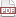### Tip 45: Structures get copied

30 December 11. [link]PDF version

level: pretty basic
purpose: get to know that pesky equals sign

Part of a series of tips on POSIX and C. Start from the tip intro page, or get 21st Century C, the book based on this series.

Copying the contents of a structure is a one-line operation:

typedef struct{
int a, b;
double c, d;
int *efg;
} demo_struct_t;

int main(){
demo_struct_t d1 = {.b=1, .c=2,
.d=3, .efg=(int[]){4,5,6}};
demo_struct_t d2 = d1;

//Let's change d1 and see if d2 changed.
d1.b=14;
d1.c=41;
d1.efg=7;

assert(d2.a==0);
assert(d2.b==1);
assert(d2.c==2);
assert(d2.d==3);
assert(d2.efg==7);
}


No, you don't get a one-line comparison function like assert(d1==d2), but the copy of one struct to another is exactly how it should be: d2 = d1.

Tip #37 advised that you should always know whether your assignment is a copy of the data or a new alias, ¿so which is it here? We changed d1.b, and d1.c and d2 didn't change, so this is a copy. But a copy of a pointer still points to the original data, so when we change d1.efg, the change also affects the copy of a pointer d2.efg.

This advises when you need a struct copying function and when it's overkill to have one and an equals sign will do.

For arrays, the equals sign will copy an alias, not the data itself. Let's try the same test of making a copy, changing the original, and checking the copy's value.

int main(){
int abc[] = {0, 1, 2};
int *copy = abc;

abc = 3;
assert(copy==3);
}


If you need a copy, you can still do it on one line, but we're back to memory-twiddling syntax:

int main(){
int abc[] = {0, 1, 2};
int *copy1, copy2;

copy1 = abc;
memcpy(copy2, abc, sizeof(int)*3);

abc = 3;
assert(copy1==3);
assert(copy2==0);
}